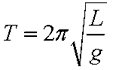SEARCH HOMEMath Central Quandaries & QueriesBarb, a student: I looked at the questions concerning a pendulum as I know I need to use this formula but I am still not able to figure this problem out. Can you help? If a child is on a swing with a 10 foot chain, then how long des it take to complete one cycle of the swing? I know I am suppose to use the formula 8T2 = pi2 L but I do not understand how to do this. ThanksHi Barb,

I assume that is a formula that is the imperial unit equivalent ofwhere T is period length in seconds, L is the length of the pendulum in metres and g is the acceleration due to gravity. This formula is much more common since it uses S.I. units (modern form of the mertric system) which are used by the scientific community.

Your formula has T, the period, which I assume is in seconds and L, the length, which I assume is in feet. You need to rearrange your expression to isolate T using basic algebra then plug in your value for length of the chain.

Hope this helps,

JaniceMath Central is supported by the University of Regina and The Pacific Institute for the Mathematical Sciences.